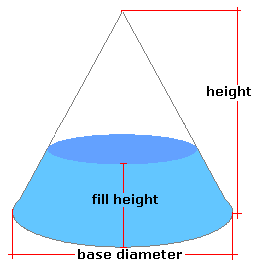Spike's Calculators

# Partial Volume in a Cone-Shape Tank/Container - FeetCalculate the amount of liquid in a partially filled conical tank or container. This calculator uses feet for measurements.

##### Approximate weight of Fuel or Water
```one US gallon of water = 8.34 pounds.
one US gallon of diesel fuel = 7.1 pounds
one US gallon of gas = 6 pounds
```

### Conical Tank

Bottom Diameter (B) ft in
Height ft in
Depth of Liquid ft in
Liquid Weight-Pounds per US Gallon lb/gal US

#### Results:

###### Tank Volume and Capacity
Cubic Feet ft³
Cubic Inches in³
Cubic Metres
Capacity in US Gallons gal US
Capacity in Litres L
###### Amount of Liquid Inside Tank
In Cubic Feet ft³
In US Gallons gal US
In Imperial Gallons gal Imperial
In Litres L
In Hectolitres hl
###### Weight of Liquid and Fill Amount
Weight of Liquid lbs
To Fill this Tank, Add gal US

#### Calculator

1. the bottom diameter (base) in feet and or inches
2. the height of the cone in feet and or inches
3. the depth of the liquid inside the tank in feet and or inches
4. the weight of the liquid in pounds per US gallons

#### Results

1. the volume of the tank in cubic feet
2. the volume of the tank in cubic inches
3. the volume of the tank in cubic metres
4. the total amount of liquid the tank can hold in US gallons
5. amount of liquid the tank can hold in litres
6. the amount of liquid inside the tank in cubic feet
7. the amount of liquid inside the tank in US gallons
8. the amount of liquid inside the tank in Imperial gallons
9. the amount of liquid inside the tank in litres
10. the amount of liquid inside the tank in hectolitres
11. the weight of the liquid inside the tank based on lbs/gal US
12. the amount of liquid it will take to fill this tank in US gallons

##### Formula
```V = 1/3𝝅(B/2)²H
where V is the volume of the cone
𝝅 = 3.14159265
B the  diameter at the base of the cone
H the height of the cone
```
##### Conversions
```one cubic foot (ft³) = 1728 cubic inches (in³)
one cubic foot (ft³) = 28316846.592 cubic millimetres (mm³)
one cubic foot (ft³) = 0.028316846592 cubic metres (m³)
one cubic foot (ft³) = 7.48051948051947 gallons (gal) US
one cubic foot (ft³) = 6.22883545904283 gallons (gal) Imperial
one square foot (ft²) = 144 square inches (in²)
one square foot (ft²) = 92903.04 square millimetres (mm²)
one square foot (ft²) = 0.09290304 square metres (m²)
```

## Cone Shaped Tank/Container#### Filled